Courses
Courses for Kids
Free study material
Offline Centres
MoreLast updated date: 23rd Nov 2023
Total views: 379.2k
Views today: 9.79k

# Draw different pairs of circles. How many points does each pair have in common? What is the maximum number of common points?Verified
379.2k+ views
Hint: In this question we need to take two circles and draw them in different positions and find how many points do they have in common. To start with this question we will take two circles apart from each other and then reduce the space between them to get another type of pair.

So, the first on would be when circles are far apart.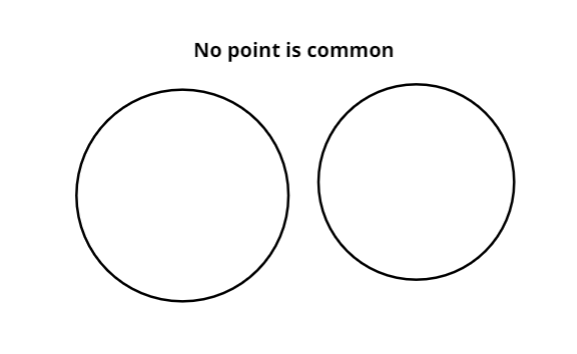As we can see in the above diagram the circles are far apart and do not touch each other so they do not have any points in common.
Now, if we decrease the distance between them and make them just touch each other we get,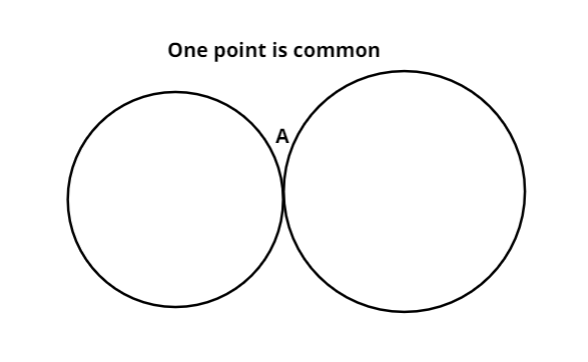So, as we can see in the above picture the circles have a common point A. Hence the number of common points for this one is one.
Now, if we further decrease the distance between them then we get,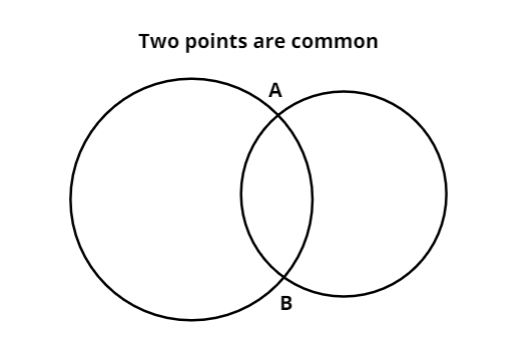So, as we can see in the above figure the circles intersect each other at A and B. So, the number of points they have in common is 2.
Now, if further decrease the distance between them we get,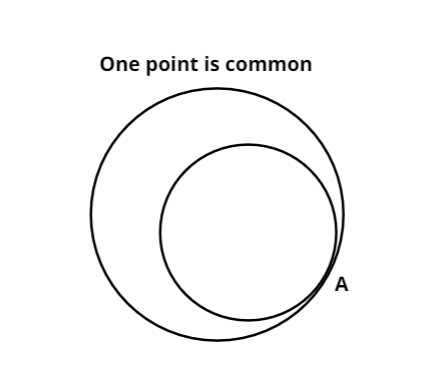So, as we can see in the above picture the circles have a common point A. Hence the number of common points for this one is one.
Now, if we further decrease the distance between them we get,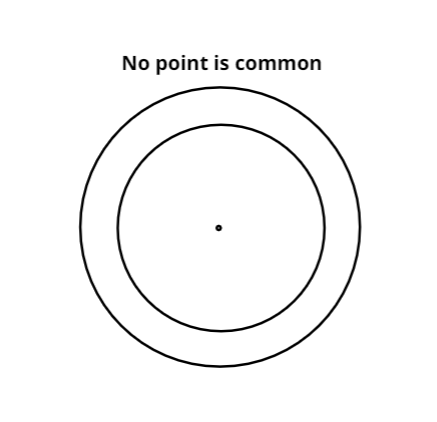So, as the above circles do not touch each other, hence there is no common point.
From the above discussion we can infer that the maximum number of common points the pair of circles can have is two.

Note: Whenever we face such types of problems the key point to remember is that we need to have a good grasp over circles and its properties. We should also try not to miss any condition as it would result in a wrong solution. This helps in getting us the required condition and gets us on the right track to reach the answer.